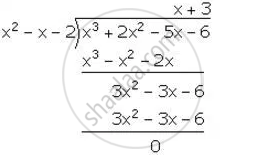Share

# If (X + 1) and (X – 2) Are Factors of X3 + (A + 1)X2 – (B – 2)X – 6, Find the Values of a and B. and Then, Factorise the Given Expression Completely. - Mathematics

Course

#### Question

If (x + 1) and (x – 2) are factors of x3 + (a + 1)x2 – (b – 2)x – 6, find the values of a and b. And then, factorise the given expression completely.

#### Solution

Let f(x) = x3 + (a + 1)x2 – (b – 2)x – 6
Since, (x + 1) is a factor of f(x).
Remainder = f(-1) = 0
(-1)3 + (a + 1)(-1)2 – (b – 2) (-1) – 6 = 0
-1 + (a + 1) + (b – 2) – 6 = 0
a + b – 8 = 0 …(i)
Since, (x – 2) is a factor of f(x).
Remainder = f(2) = 0
(2)3 + (a + 1) (2)2 – (b – 2) (2) – 6 = 0
8 + 4a + 4 – 2b + 4 – 6 = 0
4a – 2b + 10 = 0
2a – b + 5 = 0 …(ii)
Adding (i) and (ii), we get,
3a – 3 = 0
a = 1

Substituting the value of a in (i), we get,
1 + b – 8 = 0
b = 7
∴ f(x) = x3 + 2x2 – 5x – 6
Now, (x + 1) and (x – 2) are factors of f(x). Hence, (x + 1) (x – 2) = x2 – x – 2 is a factor of f(x).f(x) = x3 + 2x2 – 5x – 6 = (x + 1) (x – 2) (x + 3)

Is there an error in this question or solution?